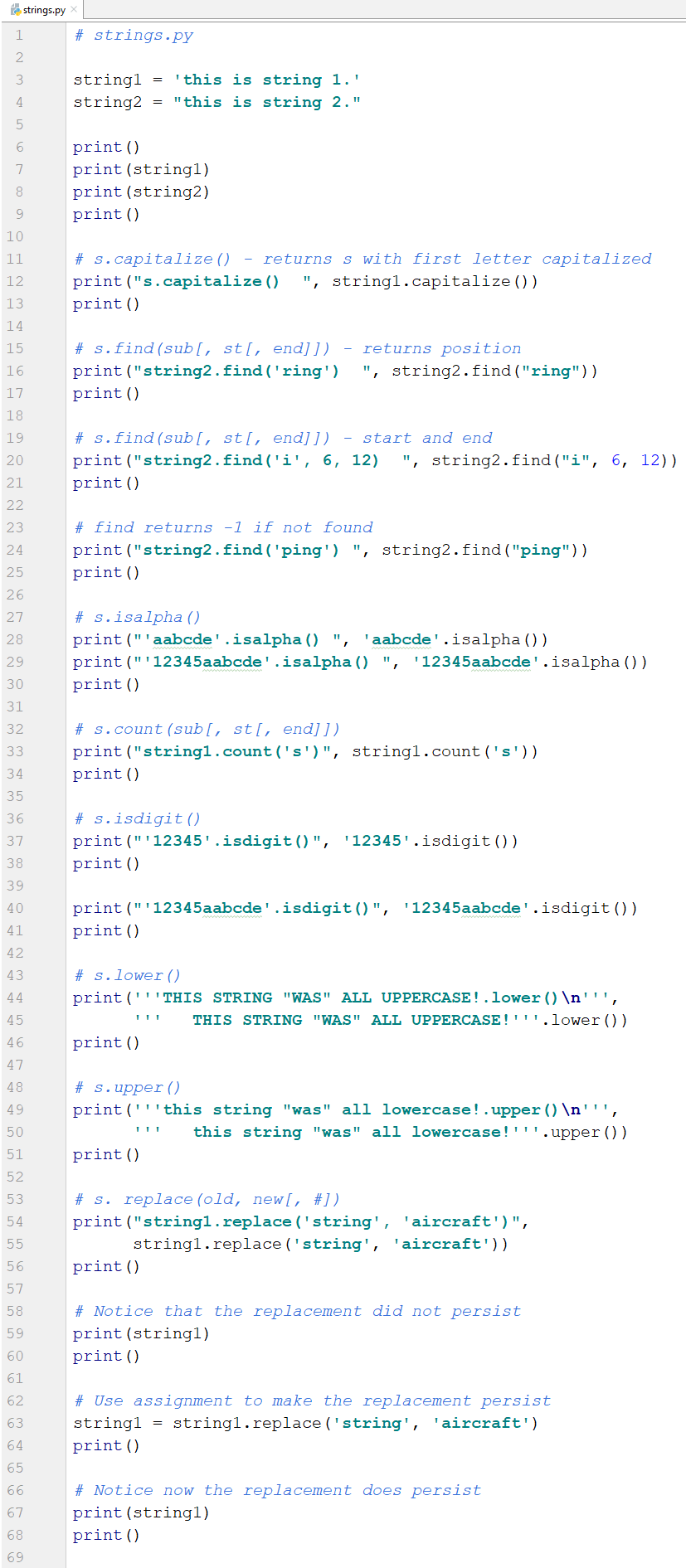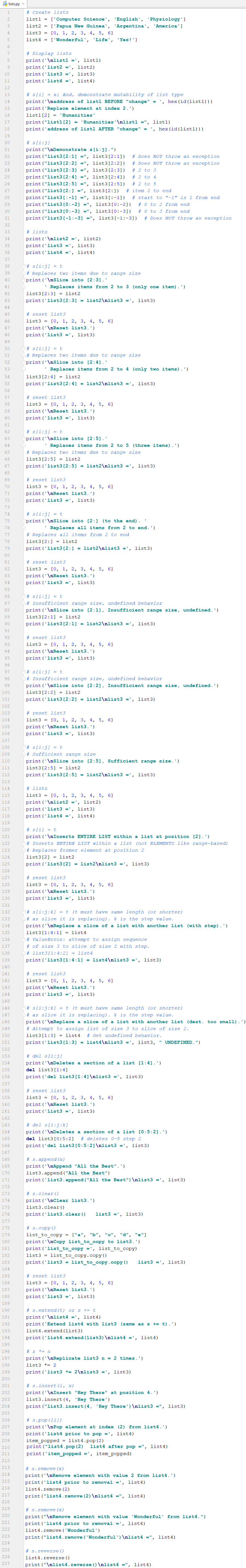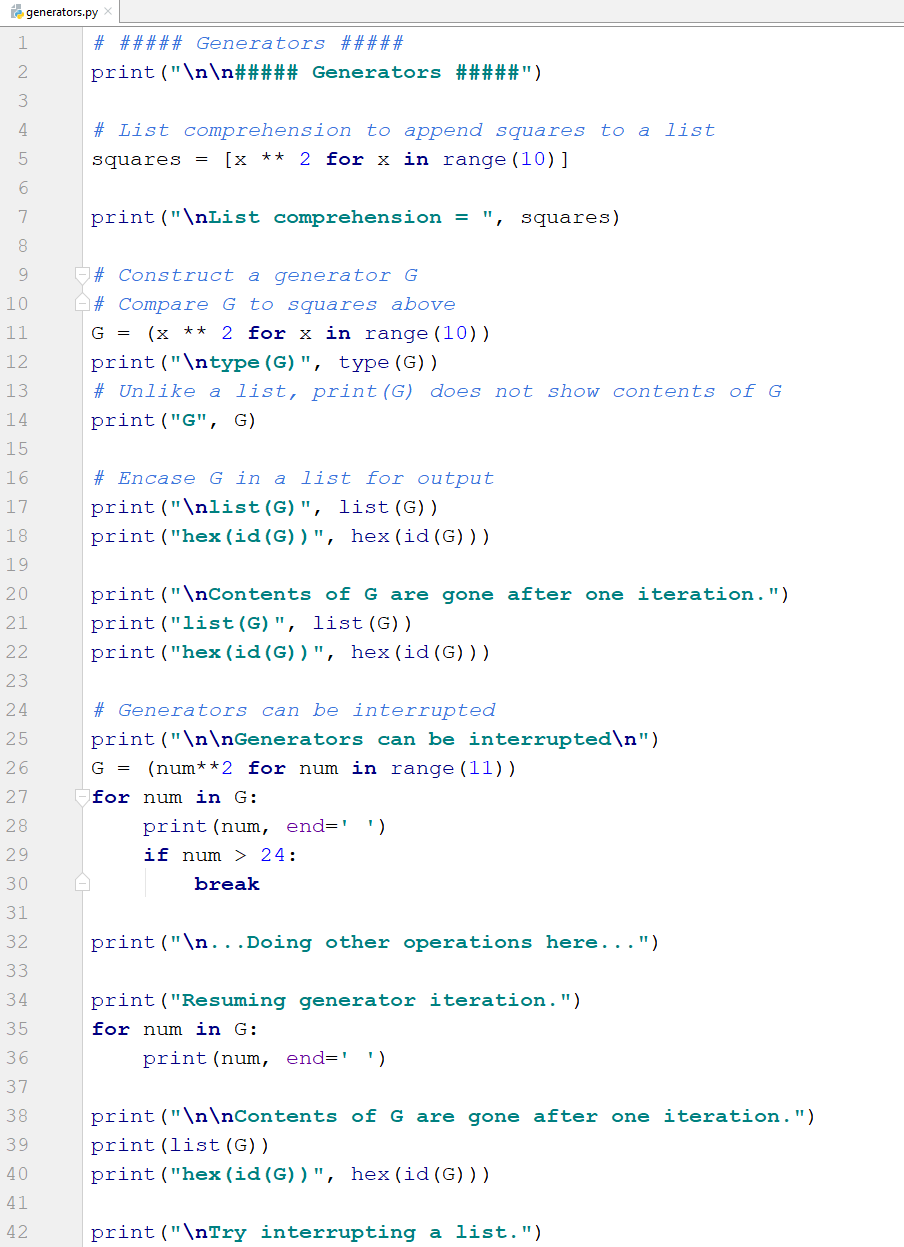# COSC 1336 | PyThon# Data Types

## Foreword

In this course, we cover the most commonly used Python data types as outlined in the Python Language Reference.

The following four general data types are discussed in the course and, unless otherwise stated, will be used in examples:

• Numbers (Integer, Boolean, Real, Complex)
• Sequences (Immutable - String, Tuple, Bytes; Mutable - Lists, Byte Arrays)
• Mappings (Dictionary)
• Sets

Tuples are created with parentheses () (and no parentheses; the default), lists are created with square brackets [], dictionaries and sets are both created with braces {}. Sequences are covered below. Students are encouraged to experiment with sequence operations using both tuples and strings.

## Data Types - Sequences

Sequences - the Python Language Reference describes sequences as finite, ordered, and indexed by non-negative numbers. This means the collections (or groups of items) are placed in order (one after another but not necessarily sorted). Each item can be accessed by an index number. Items are also known as elements. Since sequences are ordered, the contents of all sequences can be accessed with index values (a.k.a the 'ith item') via [i]. Furthermore, it is very common to use iteration and iterate over the [i] when working with sequences. Sequences come in two categories: immutable and mutable. Both types are considered below.

Note: square brackets [] have at least three meanings thus far in the course. [] are used to:

1. Access items in a sequence ([i] sequence operators)
2. Create a new list
3. Express optionality in the 'general case' (e.g. s.index(x[, i[, j]]). i and j are optional)

### Immutable Sequence Types

Let's look at the immutable sequence types first.

Immutable - sequences that cannot be changed after creation. These include strings, tuples, and bytes.

• String - a sequence of Unicode characters such as: 'abc', '123', 'Hello my name is...'.
• 128 ASCII characters; 1,112,064 Unicode possibilities
• Strings are created with single or double quotes
• Tuple - a sequence of arbitrary Python objects such as: (1, 'a', 'Greetings'). Tuples are created with ( ) or without ( ). See code.
• Bytes - a sequence of bytes (8-bits) in an immutable array represented by integers in the range of: 0<=x<256. Not covered in this course.The operations in the table below are supported by most sequence types (both mutable and immutable).

 Operations in Both Mutable and Immutable Sequences x in s True if x in s x not in s True if x not in s s + t concatenation s * n or n * s add s to itself n times s[i] ith item s[i:j] slice from i to j-1 s[i:-j] slice from i to j from end s[i:j:k] slice from i to j-1 step k len(s) length min(s) smallest item max(s) largest item s.index(x[, i[, j]]) index of first x [at or after i and before j] s.count(x) Number of x in s

In the code example below, tuples are created and used to demonstrate the operations in the table above. It should be noted that the same operations can be performed with strings.Here's the output.In addition to the operations common to all sequences listed in the table above and applied to tuples in the code listing, the string sequence type offers more options. Some of the string specific operations are shown in the following table and in the code example.

 String Operations s.count(sub[, st[, end]]) return count of sub in s s.capitalize() return s with first letter capitalized s.find(sub[, st[, end]]) find sub in s at start and until end s.isalpha() true if at least 1 char. and all alpha s.isdigit() true if at least 1 char. and all digits s.lower() converts to all lowercase s.upper() converts to all uppercase s.replace(old, new[, #]) replace old with new for # of times

The strings operations in the table are shown in the following code example.Here's the output.### Mutable Sequence Types

We have covered the immutable sequences (Strings, Tuples, and Bytes). There are also mutable sequences (Lists and Byte Arrays).

Mutable - sequences that can be changed after creation.

• List - a sequence of arbitrary Python objects such as: [1, 'a', 'Greetings'].
• Are created with square brackets [].
• Are similar to tuples but lists can be changed but tuples cannot be changed.
• Can contain items of different types but usually contain items of the same type.
• Items in a list can be accessed with index [i] values (a.k.a the 'ith item').
• Byte Arrays - a sequence with the same functionality as the bytes type except that a bytearray is a mutable array. Not covered in this course.The operations in the table below are supported only by mutable sequence types (NOT the immutable sequence types). Even though some of the operations from the Mutable Only table also appear in the Both table, the ability to modify the sequence is the distinguishing factor.

For instance, s[i:j] appears in the Both table and is only used to access/read the slice from the sequence. Whereas, in the Mutable Only table, s[i:j] can be used to access and modify the sequence content.

 Operations in Mutable Sequences Only s[i] = x item i replaced by x s[i:j] slice from i to j-1 s[i:-j] slice from i to j from end s[i:j] = t replace slice with t del s[i:j] delete slice s[i:j:k] = t elements i to j, step k replaced with t del s[i:j:k] elements i to j-1, step k are deleted s.append(x) append object x to end of sequence s.clear() deletes all elements s.copy() creates a shallow copy s.extend(t) or s += t extend s with contents (items) of t s *= n makes s its content n times s.insert(i, x) insert x at index i s.pop([i]) return and remove item i s.remove(x) remove first item x found s.reverse() reverses s

In the code example below, lists are created and used to demonstrate the operations in the table above. s.copy() is covered more thoroughly in the chapter Copy Operations. Students are encouraged to extensively experiment with sequence operations using strings, tuples, and lists.

Notice the difference between the append and extend methods in the code output. The append method appends an object to the sequence. On the other hand, the extend method adds the individual items (elements) of the object to the sequence.

Note: Slicing in Python, particularly with mutable sequences, can produce interesting and at times unexpected behavior. For instance, when attempting to slice with an irrelevant range such as s[7:3], Python does not produce an error (or throw an exception). Rather, in this case, Python (at least CPython) will insert at position 7 with no replacement. This is likely undefined behavior and should not be trusted in current or future versions or in different implementations. For more on slicing see the Python Language Reference and the Python Tutorial and search slice at the links.Here's the output.### List Comprehensions

List comprehension is a Python technique that provides a succinct method to construct lists. It uses iterators as input, supplies the iteration values to an expression, and returns a list.Here's the output.### Generators

Generators look like and iterate like list comprehensions but have the following differences:

• Use () instead of [].
• List values are stored and generator values are computed on the fly and therefore require minimal memory.
• Lists that are returned from list comprehensions have standard variable lifetime. However, generators are consumed after one iteration.
• Generators do not compute the next value until it is needed. Therefore, generators can continue infinitely.
• Generators can be interrupted and resumed.Here's the output.